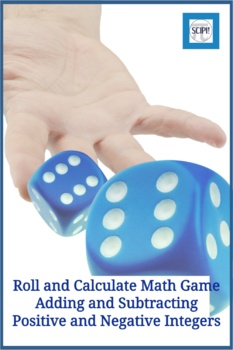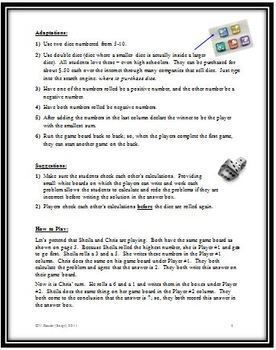# Roll and Calculate: A Math Game to Practice + and - Positive & Negative NumbersSubject
Resource Type
Product Rating
File Type

PDF (Acrobat) Document File

641 KB|6 pages
Share
Also included in:
1. This resource is a bundle of two hands-on math games that incorporate computation using positive and negative numbers. The operations of addition, subtraction and multiplication are included. The first game, Bug Mania, provides motivation for the learner to practice addition, subtraction and multipl
\$6.00
\$5.00
Save \$1.00
Product Description

Roll and Calculate is a hands-on math game designed to practice the addition and subtraction of positive and negative numbers. Two players take turns rolling two dice numbered 1-6 and then add or subtract the numbers based on the placement on the game board. The answer must be agreed upon before the next player takes his/her turn. This game is easily made more difficult by requiring the players to use dice numbered 5-10. It is a fun and interesting way for students to review adding and subtracting positive and negative numbers without doing a "drill and kill" activity.

Materials needed (two dice and the game board), complete directions, suggestions, and adaptations for the game as well as the Roll and Calculate game board are included in this five page handout.

You might also enjoy this game that uses positive and negative numbers.

Bug Mania – Two Math Games Using Positive and Negative Numbers

You can also purchase both games, Roll and Calculate and Bug Mania, in a bundle and save \$\$\$.

A Bundle of Two Math Games for Positive and Negative Numbers

You might also find these algebra resources helpful.

Order of Operations: A New Approach

Simple Math Formulas: Using a Triangular Graphic Organizer

Which Operation? Preparation Activities to Solve Math Word Problems

Total Pages
6 pages